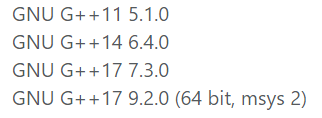# gym 102220J Time Limit

In CCPC contests, you will get “Time Limit Exceeded” when your program tried to run during too much time. Setting suitable time limit for problems is vital to a contest.

Mr. Bread is preparing problems for a coming contest with his friends. For each problem, there will be a “Main Correct Solution” denotes the standard solution program written by the author. There will also be several “Correct Solutions” denote solution programs intended to pass.

Assume there are n programs in total, labeled by 1,2,…,n. The 1-th program denotes the “Main Correct Solution” while others are “Correct Solutions”. The i-th program runs in ai seconds.

According to the rules in Mr. Bread’s mind, the time limit x should meet all the rules below:

The constraint can’t be too tight, which means x≥3a1.
All the “Correct Solutions” should pass, which means x≥ai+1 for all i∈[2,n].
x should be the smallest even integer meeting the rules described above.
Please write a program to find the time limit x.

Input
The first line of the input contains an integer T(1≤T≤10), denoting the number of test cases.

In each test case, there is one integer n(2≤n≤10) in the first line, denoting the number of programs.

In the second line, there are n integers a1,a2,…,an(1≤ai≤10).

Output
For each test case, print a single line containing an integer, denoting the value of x.

Example
Input
2
2
1 3
2
1 4
Output
4
6

#include <cstdio>
#include <cstring>
#include <cstdlib>
#include <iostream>
#include <algorithm>
using namespace std;

int main()
{
int t, n, i;
int a;
int maxx;
scanf("%d\n", &t);
while (t--)
{
maxx = -1;
scanf("%d", &n);
scanf("%d", &a);
a = 3 * a;
for (i = 2; i <= n; i++) {
scanf("%d", &a[i]);
a[i] += 1;
}
for (i = 1; i <= n; i++) {
maxx = maxx > a[i] ? maxx : a[i];
}
if (maxx % 2 != 0)
maxx++;
printf("%d\n", maxx);
}
return 0;
}01-2011-1758
11-09728
12-22
03-05
10-23362
08-101615
07-0773
06-21100